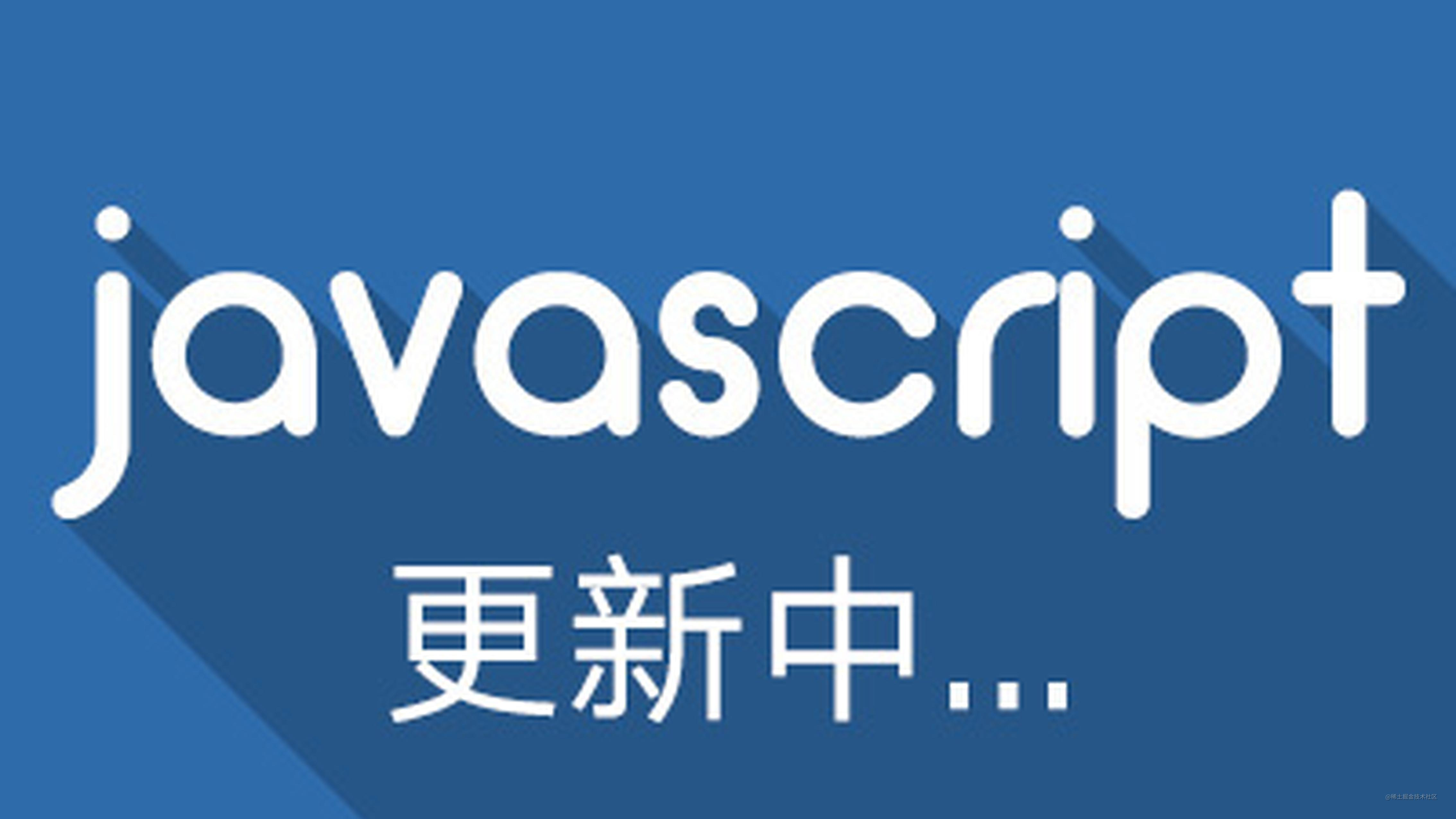# 面试20个人居然没有一个写出数组扁平化?如何手写flat函数# 实现扁平化的方法 封装 flatten

## 1. ES6 flat

flat(depth) 方法会按照一个可指定的深度递归遍历数组，并将所有元素与遍历到的子数组中的元素合并为一个新数组返回。

`depth`(可选) 指定要提取嵌套数组的结构深度,默认值为 1

`````` const arr = [1, [2, [3, [4, 5]]], 6]

function flatten(params) {
return params.flat(Infinity)
}
console.log(flatten(arr));

// 输出: [1,2,3,4,5,6]

## 2. toString

``````function flatten(arr) {
return arr.toString().split(',').map(item =>parseFloat(item))
}
console.log(flatten(arr));
// 输出:[ 1, 2, 3, 4, 5, 6 ]

## 3. 使用正则替换

``````function flatten (arr) {
console.log('JSON.stringify(arr)', typeof JSON.stringify(arr))
let str= JSON.stringify(arr).replace(/(\[|\])/g, '');
str = '[' + str + ']';
arr = JSON.parse(str);
return arr
}
console.log(flatten(arr))

**得分 : **⭐ (并不是要考你的数组的方法调用)

## 4. 循环递归

### 4.1 循环 + concat + push

` [1, [2, 3,4,5,6]]`

``````let result = [];
for (let i = 0; i < arr2.length; i++) {

result = result.concat((arr2[i]));

}

console.log(result);
[ 1, 2, 3, 4, 5, 6 ]

1. 循环判断数组的每一项是否是数组: `Array.isArray(arr[i])`
2. 是数组就递归调用上面的扁平化一层的代码 `result = result.concat(flatten(arr[i]));`
3. 不是数组,直接通过push添加到返回值数组
``````function flatten(arr) {
let result = [];
for (let i = 0; i < arr.length; i++) {
if (Array.isArray(arr[i])) {
result = result.concat(flatten(arr[i]));
} else {
result.push(arr[i])
}
}
return result
}

console.log(flatten(arr));

``````// 递归版本的反嵌套
function flatten(array) {
var flattend = [];
(function flat(array) {
array.forEach(function(el) {
if (Array.isArray(el)) flat(el);
else flattend.push(el);
});
})(array);
return flattend;
}

### 4.2 增加参数控制扁平化深度

``````// forEach 遍历数组会自动跳过空元素
const eachFlat = (arr = [], depth = 1) => {
const result = []; // 缓存递归结果
// 开始递归
(function flat(arr, depth) {
// forEach 会自动去除数组空位
arr.forEach((item) => {
// 控制递归深度
if (Array.isArray(item) && depth > 0) {
// 递归数组
flat(item, depth - 1)
} else {
// 缓存元素
result.push(item)
}
})
})(arr, depth)
// 返回递归结果
return result;
}

// for of 循环不能去除数组空位，需要手动去除
const forFlat = (arr = [], depth = 1) => {
const result = [];
(function flat(arr, depth) {
for (let item of arr) {
if (Array.isArray(item) && depth > 0) {
flat(item, depth - 1)
} else {
// 去除空元素，添加非 undefined 元素
item !== void 0 && result.push(item);
}
}
})(arr, depth)
return result;
}

### 4.3 巧用 reduce

reduce 方法为数组中的每个元素按序执行一个`reducer`函数,每一次运行 reducer 会将先前元素的计算结构作为参数传入,最后将其结果汇总为单个返回值

1. `callbackFn` 一个 reducer 函数,包含四个参数:
• previousVal :上一次调用`callbackFn`时的返回值,在第一次调用时,若指定了初始值`initialValue`,previousVal 的值就位 initialValue,否则初始值就是为数组的索引为 0 的元素
• `currentVal`:数组中正在处理的元素,在第一次调用时,若指定了初始值,其值则为数组索引为 0 的元素 array,否则为 array
• currentIndex: 数组中正在处理的元素的索引,若指定了初始值 initialValue,则起始索引号为 0,否则从索引 1 起始
• array : 用于遍历的数组
1. `initialValue`(可选) : 作为第一次调用 callback 函数时参数 previousValue 的值

``````const arr = [1, [[2, 3], 4],5]
const result = arr.reduce((acc, val) => acc.concat(val), []);
console.log(result);
// 输出: [1,[2,3],4,5]

``````const arr = [1, [[2, 3], 4],5]
const flatten = (arr, deep = 1) => {
if (deep <= 0) return arr;
return arr.reduce((res, curr) => res.concat(Array.isArray(curr) ? flatten(curr, deep - 1) : curr), [])
}
// function flatten (arr,deep=1) {
// return   arr.reduce((acc,val) => acc.concat(Array.isArray(val)? flatten(val,deep-1):val),[])
// }
console.log(arr, Infinity);
// 输出:[ 1, 2, 3, 4, 5, 6 ]

### 4.4 使用 `Generator` 函数

GeneratorFunction是协程在 ES6 的实现，最大特点就是可以交出函数的执行权（即暂停执行）。

``````function* flatten(array) {
for (const item of array) {
if (Array.isArray(item)) {
yield* flatten(item);
} else {
yield item;
}
}
}

## 5. 使用堆栈 stack 避免递归

1. 把数组通过一个栈来维护
2. 当栈不为空的时候循环执行处理
3. pop()将栈尾出栈
4. 如果出栈的元素是数组,就将该元素解构后每一元素进行入栈操作
5. 出栈的元素不是数组就push进返回值res
6. 反转恢复原数组的顺序
``````var arr1 = [1,2,3,[1,2,3,4, [2,3,4]]];
function flatten(arr) {
const stack = [...arr];
const res = [];
while (stack.length) {
// 使用 pop 从 stack 中取出并移除值
const next = stack.pop();
if (Array.isArray(next)) {
// 使用 push 送回内层数组中的元素，不会改动原始输入
stack.push(...next);
} else {
res.push(next);
}
}
// 反转恢复原数组的顺序
return res.reverse();
}
flatten(arr1);// [1, 2, 3, 1, 2, 3, 4, 2, 3, 4]

## 6.while 循环+ some方法

some :方法测试数组中是不是至少有 1 个元素通过了被提供的函数测试。它返回的是一个 Boolean 类型的值。

``````function flatten(arr) {
while (arr.some(item=> Array.isArray(item))) {
console.log(...arr)
arr = [].concat(...arr)
console.log(arr)
}
return arr
}
console.log(flatten(arr));